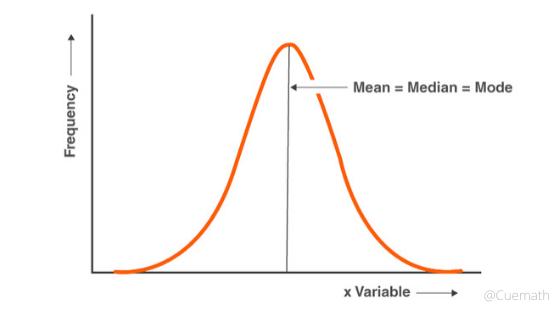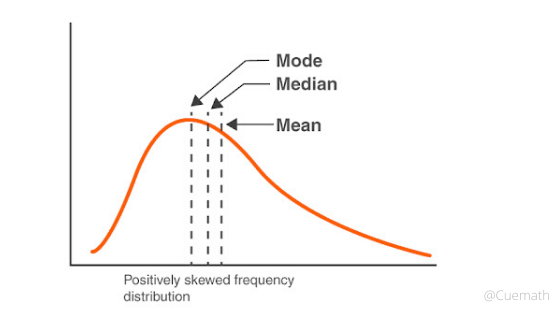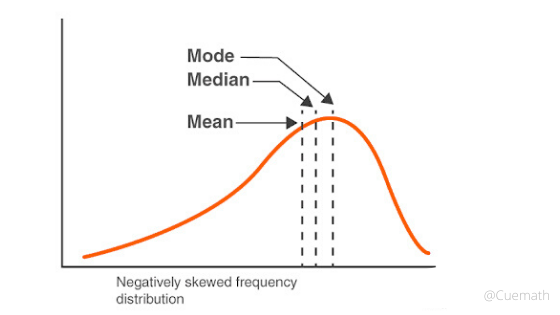Math Concepts

# Mean, Median and Mode: Understanding the relation between them

63.1k views

 1 Introduction 2 What is Mean? 3 What is Median? 4 What is Mode? 5 Relationship Between Mean, Median, and Mode 6 Summary 7 Frequently Asked Questions (FAQs) 8 External References

04 January 2021

What is average?

What is arithmetic mean?

To understand the concept of mean, median, and mode, the relationship between them, and the difference, we first need to know that these concepts form the part of measures of central tendency.

It refers to a single value that attempts to describe the characteristic of the entire set of data by identifying the central position within that set. It is sometimes also known as measures of central location. Colloquially, measures of central tendency often referred to only averages. But now we have a lot more than averages.

The most common measures of central tendency are the mean, the median, and the mode. We have to remember that the measure of central tendency is generally performed on a finite set of values.

The concept of mean, median, and mode and their relationship is briefly explained in the below Downloadable PDF. Click on the download button to view this.

## What is Mean?

By ‘mean’ we refer to an Arithmetic mean. There are other types of ‘mean’ like geometric mean and harmonic mean which is out of the scope of this article.

The arithmetic mean refers to the average of a data set of numbers. It can either be a simple average or a weighted average.

To calculate a simple average, we add up all the numbers given in the data set and then divided by how many numbers there are.

Why do we need to analyze this relation?

It is important to understand the concepts and the relation between these terms so that we can apply them in real life for statistics and analytics. For example, How is math used in football?

Example: What is the mean of 6, 7, and 11?

Divide by how many numbers (i.e. 3 numbers): 24 ÷ 3 = 8

So the arithmetic mean = 8.

To calculate the weighted average, we multiply the given numbers with the required weights and divide the result by the sum of the weights.

## What is Median?

Median is the middle number of a given data set when it is arranged in either a descending order or in ascending order.

If there is an odd amount of numbers, the median value is the number that is in the middle whereas if there is an even amount of numbers, the median is the simple average of the middle pair in the dataset. Median is much more effective than a mean because it eliminates the outliers.

Example: Find the median of the data set {3, 13, 2, 34, 11, 26, 47}.

Answer: When sorted in the ascending order, it becomes {2, 3, 11, 13, 26, 34, 47}.

The amount of numbers in the data set is seven which is odd.

Therefore, the median is the number in the middle, which in this instance is 13.

## What is Mode?

The mode refers to the number that appears the most in a dataset. A set of numbers may have one mode, or more than one mode, or no mode at all.

Example: Find the mode of the following set of scores {14 11 15 9 11 15 11 7 13 12}.

Answer: The mode is 11 because 11 occurred more times than the other numbers.

So, as individually explained, these were some of the basic measures of central tendency. As you can see, there is a huge difference between mean, median, and mode.

But, there is also a relationship between mean, median, and mode. We term this relationship as the empirical relationship between mean, median, and mode.

## Relationship Between Mean, Median, and Mode

We will understand the empirical relationship between mean, median, and mode by means of a frequency distribution graph. We can divide the relationship into four different cases:

1. In the case of a moderately skewed distribution, i.e. in general, the difference between mean and mode is equal to three times the difference between the mean and median.

Thus, the empirical relationship as Mean – Mode = 3 (Mean – Median).

1. In the case of a frequency distribution that has a symmetrical frequency curve, the empirical relation states that mean = median = mode.1. In the case of a positively skewed frequency distribution curve, mean > median > mode.1. In the case of negatively skewed frequency distribution mean < median < mode.### Let us continue understanding the relationship between mean, median, and mode formula with the help of an example.

Example: It is given that in a moderately skewed distribution, median = 10 and mean = 12. Using these values, find the approximate value of mode.

Answer: Let us take mode to be ‘x’. We have been given that the median = 10 and mean = 12. Now, using the relationship between mean mode and median we get, (Mean – Mode) = 3 (Mean – Median)

So, 12 – x = 3 (12 – 10)

12 – x = 3*2

12 - x = 6

-x = -6 or x = 6

So, Mode = 6

You may also like:

## Summary

In statistics, Mean, Median, and Mode are typical values to represent a pool of numerical observations. They are calculated from the pool of observations.

• Mean is the average of all the values.
• Median is the middle value, dividing the number of data into 2 halves. In other words, 50% of the observations is below the median and 50% of the observations are above the median.
• Mode is the most common value among the given observations.

Cuemath, a student-friendly mathematics and coding platform, conducts regular Online Live Classes for academics and skill-development, and their Mental Math App, on both iOS and Android, is a one-stop solution for kids to develop multiple skills. Understand the Cuemath Fee structure and sign up for a free trial.

## How do you find the mean median and mode?

Arrange data points from smallest to largest and locate the central number. This is the median. If there are 2 numbers in the middle, the median is the average of those 2 numbers. The mode is the number in a data set that occurs most frequently.

## What is a range in mean median mode?

A Range is a difference between two numbers.

## External References

Related Articles
GIVE YOUR CHILD THE CUEMATH EDGE
Access Personalised Math learning through interactive worksheets, gamified concepts and grade-wise courses